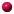#Applied Physics Photo Courtesy Mark Meier - So There!

## Problem Statement

We can apply the principles described on the Physics Page to figure out the motor needed for your rocket and how fast your rocket will be going when it reaches the end of the launch rod.

These two questions are closely related, because the thrust requirement for your rocket is that the thrust has to be high enough to get your rocket going fast enough to be aerodynamically stable when it comes off the launch rod. To meet that condition, your rocket needs to be going about 10 meters per second (or faster) when it reaches the end of the launch rod. If the launch rod is the typical length of 1 meter, then the problem statement comes down to

How much thrust is needed to accelerate to 10 m/s within a distance of 1 meter?

## Solving the Problem

We will solve the problem in the general case so you can find the right value for any launch rod length. To solve this problem we need an expression for velocity as a function of distance (or vice versa) while under constant acceleration. So let's take a look at what happens while the rocket's accelerating.

• The rocket starts at altitude = 0, velocity = 0.

• After accelerating for time t, with acceleration a, the final velocity is v = a*t. The velocity progressively increases from zero to this final value. So after half the time, the velocity has increased to v = 0.5*a*t. For half the time it was going slower than 0.5*a*t, and for half the time it's going faster. On the average, then, the rocket travels at v = 0.5*a*t.

• We can use the average value of velocity to figure out the distance traveled during the acceleration time t. Since distance s = v*t, and v = 0.5*a*t, then

s = 0.5*a*t2

This is the basic physics equation for distance covered under constant acceleration.

Now lets substitute the acceleration & velocity equation in order to get the relationship between velocity, acceleration, and distance:

v = a*t
t = v/a

s = 0.5*a*t2 = 0.5*a*(v/a)2 = 0.5*(v2)/a = (v2)/(2*a)

We can change the form to something more useful,

a = (v2)/(2*s)

Then substitute a form of Newton's Second Law,

a = F/m = (v2)/(2*s)

For our purposes we're only going to be worried about relatively low velocities and hence drag is not a significant contributor. Then the force on the rocket is just the motor thrust minus the weight of the rocket. Note that the weight of the rocket in Newtons is the mass of the rocket in kilograms (=mass in grams/1000) multiplied by the acceleration of gravity, or g = 9.8 m/s/s. Then our equation becomes:

(T - m*g)/m = (v2)/(2*s)

where T is the motor thrust that we want to find.

Rearranging leads us to the final form of the equation as we will use it:

T/m = (v2)/(2*s) + g

Where

• T is the motor thrust in Newtons
• m is the rocket mass (with full engine mass) in kilograms
• v is the speed we want at the end of the launch rod (10 m/s)
• s is the length of the launch rod (around 1-2 meters)
• g is the acceleration of gravity = 9.8 m/s/s

## Some Examples

So why, you may well ask, did I set up the equation to compute "T/m", the thrust to mass ratio, rather than computing the thrust? Let's try an example and you'll see why this is a good way to do it.

Let's stipulate that we're launching model rockets (low power) and using a 39" launch rod (that is, one meter long). Then our equation works out to be

T/m = (v2)/(2*s) + g = (102)/(2*1) + 9.8 = 59.8 N/kg

Thanks to Paul Mansbacher for the correction.

That's 59.8 Newtons of thrust for every kilogram of rocket. The thing is, no one measures their Estes Alpha rocket in kilograms. Normally we talk about the rocket mass in ounces or grams.

The conversion from Newtons/kilogram to Newtons/gram is easy, if you need 59.8 N for a kilogram, you need 1/1000 of that for a gram, or 0.0598 N/g. Since there are 35.27 ounces per kilogram, then you can find the value for ounces as 59.8/35.27 = 1.7 Newtons/ounce.

So for example, since my Astrocam is 3.7 ounces (without the motor), it requires a minimum thrust of 3.7*1.7 = 6.3 Newtons. No wonder the recommended motor for this rocket is a C6-7 - ONLY (see note below).

Notice that we now have a number that applies to any rocket launching from a 1 meter launch rod. Once we have the conversion factor of, say, 1.7 N/oz, we can find the minimum thrust for any rocket by multiplying its mass in ounces by 1.7.

If I'm launching high power rockets with a 2-m launch rod, the figure is then

T/m = (102)/(2*2) + 9.8 = 34.8 N/kg = 15.8 N/lb

So my Minie Magg, which is 2.5 lbs., needs a minimum thrust of 2.5*15.8 = 39.5 Newtons when launching off a two-meter rod. I'll leave it as an exercise for the reader to figure out what the minimum thrust is to launch from a four-foot (1.22 meter) launch rod.

Note on the Astrocam Example:Back to Thrust & Mass StudiesBack to Rocket SimulationBack to Rocket Equations

## Questions

Your questions and comments regarding this page are welcome. You can e-mail Randy Culp for inquiries, suggestions, new ideas or just to chat.
Updated 24 August 2008